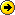# CONFIG-REGISTER

To change the the config register you need to turn on bit 6 with 0x2142 instead of default 0x2102.How does the 2142 relate to bit 6.

•www.techexams.net/technotes/ccna/basic_router_management.shtml

Also check out the link in it to the meaning of the bits settings.

You've got 16 bits in the config-register. Counting from the right - the "6th" bit is actually the 7th bit from the right, since the numbering starts with zero.

The 16 bits are represented in hex, so take them in groups of 4 = a nibble.

The "6th" bit is in the 2nd nibble from the right and has a value of 4 when you turn it on, so when the 2nd nibble from the right has a value of 4, then you know the "6th" bit is on.

So... 0x2142 would actually be: 0010000101000010 ...
where you have the 2nd, 7th, 9th, and 14th bit from the right turned on - which are actually the 1st, 6th, 8th, and 13th positions.

Make sense?

-Mike
• mwgood wrote:

You've got 16 bits in the config-register. Counting from the right - the "6th" bit is actually the 7th bit from the right, since the numbering starts with zero.

The 16 bits are represented in hex, so take them in groups of 4 = a nibble.

The "6th" bit is in the 2nd nibble from the right and has a value of 4 when you turn it on, so when the 2nd nibble from the right has a value of 4, then you know the "6th" bit is on.

So... 0x2142 would actually be: 0010000101000010 ...
where you have the 2nd, 7th, 9th, and 14th bit from the right turned on - which are actually the 1st, 6th, 8th, and 13th positions.

Make sense?

-Mike
Thank's for the reply i was missing the zero in the count.Thank's again and good luck with the ccna.